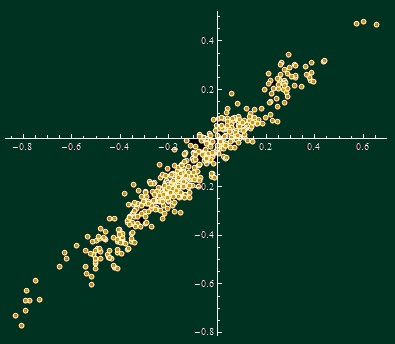## Friday, September 03, 2010

### The likelihood that 2010 will surpass 1998

It's time for some climate change facts. This text is just an update of an article written two months ago in which I add two additional months of data and refresh all the numbers.

The nearly final temperature readings of UAH AMSU for August 2010 are out and the global temperature anomaly was 0.51 °C.So August 2010 was 0.01 °C cooler than August 1998 and it is an average month of 2010 so far. Also, the average anomaly of the first eight months (Part1) of 2010, 0.55 °C, is cooler than Part1 of 1998, 0.61 °C, by 0.06 °C. Of course, if this temperature difference continued for the last four months (Part2) of 2010, 2010 would be cooler than 1998.

But Part2 is different from Part1, so this simple extrapolation isn't possible. You may ask: how likely it is for 2010 to be cooler than 1998 if we know that Part1 of 2010 was 0.06 °C cooler than Part1 of 1998? To answer this question statistically, let's simply look at 31 years of the UAH AMSU record, 1979-2009.

We may look at (31 choose 2) = 465 pairs of years. For each of them, we may calculate the difference of the average temperatures of their first 8 months, Part1, and the difference of the average annual temperatures. The Part1 differences will be shown on the x-axis and the annual (2 Part1 + Part2)/3 differences will appear on the y-axis of the chart below:A pretty nice, straight line with a pretty nice, normal "noisy" distribution away from the line itself. It's of course more straight than what we obtained two months ago: our ability to predict the average of 2010 is getting better as we approach the last month. Linear regression gives
yearDifference = -0.031 + 0.87 h1Difference
Note that the slope, 0.87, is positive but smaller than one. We may say that while Part2 tends to be correlated with Part1, Part2-Part1 is actually negatively correlated with Part1 itself, so Part2 acts to reduce the temperature difference between two years in most cases.

Now, the individual data points deviate from the idealized line. What is the root mean square of the differences? The answer turns out to be 0.055 °C (less than 0.073 °C we got two months ago). This is the "thickness" of the line in the chart above in the y-direction.

It's enough for us to make a prediction for 2010, using the comparison with 1998.

The Part1 was 0.063 °C cooler than in 1998. Multiply it by the slope, 0.87, to see that the whole year 2010 is predicted to be 0.054 °C cooler than the year 1998. At least, that's the central value. However, this predicted difference has some normally distributed noise whose standard deviation is 0.055 °C, as we said.

That means that the predicted difference is 0.98 standard deviations (0.054/0.055). Use the error function, or (approximately) this graph,to see that the probability that 2010 will still end up being warmer than 1998, according to UAH AMSU, is about
P = 16 percent.
The formula in Mathematica is (1 + Erf[-0.98/Sqrt])/2. The odds are about 5-to-1 that 2010 will stay cooler than 1998. Five is kind of greater than one - but it is not "overwhelmingly" greater - so I would agree with Roy Spencer's conclusion that "it is too close to call".

This time, I will omit the alternative ways to predict 2010 from a smaller (or weighted) ensemble of the months.

If 1998 remains the warmest year on record, you may wonder what is the appropriate global warming definition or the meaning of global warming that is consistent with the likely fact that 1998 will fail to be surpassed 12 times in a row.

#### 1 comment:

1.I did a bit of this kind of forecasting last year when I was betting on whether 2009 would beat 1998. I used the following method with HADCRUT data going back 160 years or so; summarized here.

I just ran OLS where the dependent variable was the average temperature for the year, independent variables were a pile of lags and the monthly average temperatures for the months experienced so far in the year being predicted. Get a predicted value for each year's average temperature, including current year. Get the difference between the current year's predicted value and the temperature you'd need to beat 1998. Then, count the fraction of times the absolute value of the residual exceeded that value.